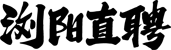• 登录 | 注册• 不限
• 市场销售
• 行政人事
• 城建装修
• 客户服务
• 会计财务
• 普工技工
• 文体培训
• 文职文员
• 设计包装
• 百货零售
• 工厂工业
• 餐饮休闲
• 金融保险
• 管理运营
• 物流贸易
• 司机后勤
• 网络硬件
• 机械仪表
• 咨询顾问
• 电子通讯
• 医疗美容
• 翻译法律
• 轻工工艺
• 化工制药
• 摄影影视
• 能源环保
• 编辑发行
• 其他分类

• 不限
• 财务主管/经理
• 会计
• 会计助理
• 注册会计师
• 注册审计师
• 审计
• 总账主任
• 出纳
• 审计(核)主管
• 统计
• 财务分析
• 成本分析/核算
• 帐目(进出口)管理
• 营运会计经理
• 审计会计经理
• 会计财务其他相关职位

• 浏阳城区
• 浏阳经开区/洞阳
• 浏阳高新区/永安
• 浏阳市两型产业园/古港
• 大瑶
• 文家市
• 金刚
• 中和
• 澄潭江
• 镇头
• 官桥
• 普迹
• 枨冲
• 柏加
• 葛家
• 北盛
• 蕉溪
• 沙市
• 社港
• 龙伏
• 淳口
• 沿溪
• 达浒
• 官渡
• 大围山
• 永和
• 张坊
• 小河
• 高坪

• 不限
• 今天
• 三天内
• 一周内
• 一个月内
• 三个月内

• 不限
• 全职
• 兼职

• 不限
• 1000元以下
• 1000-2000元
• 2000-3000元
• 3000-5000元
• 5000-8000元
• 8000-1.2万元
• 1.2万-2万元
• 2万元以上
• 面议